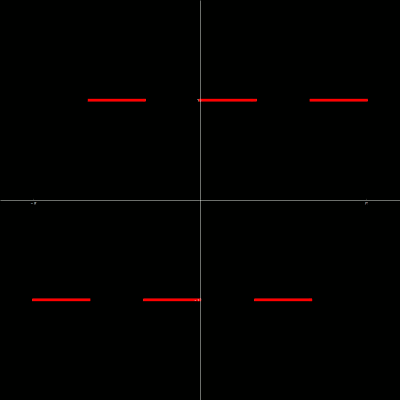M A T H 2 1 B
Mathematics Math21b Spring 2017
Linear Algebra and Differential Equations
Linear algebra Math21b, Spring 2017
 The exams page has now the exam and a solution draft. The exhibits page has more entries like the Determinant sticker or Pascal matrices which appeared in the Final exam [PDF]. The following handouts are crucial for the last part of the course The pendulum (animated below in Javascript) is simplified from a double pendulum. It is a periodically driven pendulum with friction x''=-sin(x)+ c x' + a sin(t) a system which is already chaotic in certain domains.We are almost done with Math 21b. If you are thinking about future courses, the good news is that having done 21a and 21b essentially opens up all 100 level courses at the Math, the Stats and Applied Math departments. Also more physics, more advanced economics, computer science and chemistry courses will make sense with math 21a/b under the belt. In general, course choice is a highly personal matter. Advise which is good for one student might not be optimal for an other. Here are some examples to see what courses you could take later. The choice of examples is by no means a recommendation, nor does it mean that anything not mentioned should be avoided. Courses change from semester to semester and about matter of taste it is hard to advise "de gustibus non est disputandum" and there are always also new courses coming in or old courses disappear or change. Mathematics courses sample ```- Math 101 is an intro course to proofs - Math 112 real analysis "calculus with proofs". Can also serve as an intro to proofs) - Math 114 measure, integration and banach spaces. Functional analysis - Math 115 methods of analysis, useful for physics, requires Math 112. - Math 116 real analysis, convexity and optimization - Math 118 introduces dynamical systems no preknowledge in proofs required - Math 121 linear algebra with proofs, a more abstract, axiomatic approach - Math 122 theory of groups and vector spaces - Math 131 topology, generalizes geometry to much more general spaces - Math 136 differential geometry, develops calculus on curved spaces like surfaces - Math 152 discrete math, usually taught in a seminar format - Math 154 a basic introduction into probability theory - Math 155 combinatorics, counting techniques and methods in finite mathematics. - Math 157 Math in the world, no proofs, focus on problem solving ``` Statistics courses sample ```- Stat 110 Probability, a basic introduction into probability theory - Stat 111 Theoretical statistics - Stat 115 Computational biology and bioinformatics - Stat 121 Introduction to data science - Stat 132 Applied quantitative finance - Stat 139 Statistical sleuthing through linear models - Stat 140 Design of experiments - Stat 171 Introduction to Stochastic Processes ``` Applied math courses sample ```- AM 104 does more Fourier stuff and lots more other things - AM 105 more differential equations both ODEs and PDEs. - AM 108 is nonlinear dynamical systems - AM 115 is modeling real-life phenomena - AM 120 applied linear algebra and big data - AM 121 optimization some Markov chains dynamical systems among other things - AM 158 feedback control systems geared towards engineering ``` Computer Science courses sample ```- CS 20 Discrete Mathematics for Computer Science - CS 50 Introduction to Computer science - CS 51 Introduction to Computer Science II - SC 109 Introduction to Data Science - CS 121 Introduction to Computation - CS 124 Data Structures and Algorithms - CS 125 Algorithms and Complexity - CS 127 Cryptography - CS 134 Networks ``` Physics courses sample ```- Physics 15a Mechanics Relativity - Physics 15b Electromagnetism Stats - Physics 15c Wave phenomena - Physics 16 Mechanics and relativity - Physics 143 Quantum mechanics - Physics 151 Mechanics - Physics 153 Electrodynamics - Physics 181 Statistical Mechanics and Thermodynamics - Physics 195 Solid state physics ``` Economics courses samples ```- Econ 1034 Networks - Econ 1051 Introduction to Game theory - Econ 1123 Introduction to Econometrics - Econ 1126 Quantitative methods in Economics ```